# GMAT Math : Graphing inverse variation

## Example Questions

### Example Question #1 : How To Graph Inverse Variation

Give the vertical asymptote of the graph of the equation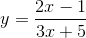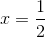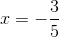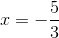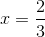Explanation:

The vertical asymptote is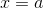, whereis found by setting the denominator equal to 0 and solving for: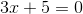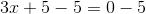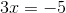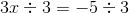This is the equation of the vertical asymptote.

### Example Question #2 : How To Graph Inverse Variation

Give the-intercept(s), if any, of the graph of the equation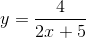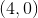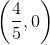The graph has no-intercept.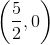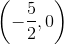The graph has no-intercept.

Explanation:

Set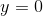in the equation and solve for.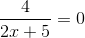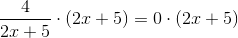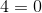This is impossible, so the equation has no solution. Therefore, the graph has no-intercept.

### Example Question #3 : How To Graph Inverse Variation

Give the-intercept(s), if any, of the graph of the equation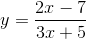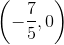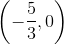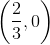The graph has no-intercept.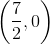Explanation:

Setin the equation and solve for.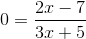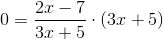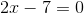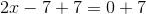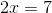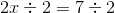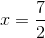The-intercept is### Example Question #4 : How To Graph Inverse Variation

Give the horizontal asymptote, if there is one, of the graph of the equation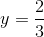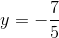The graph of the equation has no horizontal asymptote.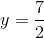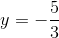Explanation:

To find the horizontal asymptote, we can divide both numerator and denominator in the right expression by: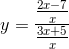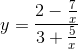Asapproaches positive or negative infinity,and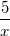both approach 0. Therefore,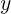approaches, making the horizontal asymptote the line of the equation.

### Example Question #5 : How To Graph Inverse Variation

Give the-intercept of the graph of the equation.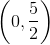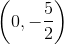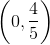The graph has no-intercept.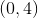Explanation:

Setin the equation: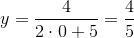The-intercept is.

Tired of practice problems?

Try live online GMAT prep today.